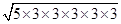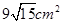### Herons Formula - Solutions -

CBSE Class 9 Mathematics

NCERT Solutions
CHAPTER 12
Herons Formula(Ex. 12.1)

1. A traffic signal board, indicating ‘SCHOOL AHEAD’ is an equilateral triangle with side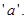Find the area of the signal board, using Heron’s formula. If its perimeter is 180 cm, what will be the area of the signal board?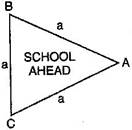Ans.
Let the Traffic signal board is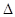ABC.

According to question, Semi-perimeter ofABC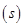=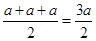Using Heron’s Formula, Area of triangle ABC =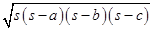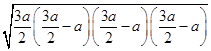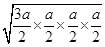=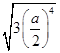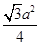square units

Now, Perimeter of this triangle = 180 cm

$⇒$ 3 x Side of triangle $\left(a\right)$ = 180 cm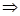Side of triangle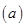=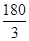= 60 cmSemi-perimeter of this triangle =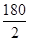= 90 cm

Using Heron’s Formula, Area of this triangle =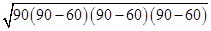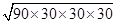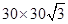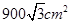2. The triangular side walls of a flyover has been used for advertisements. The sides of the walls are 122 m, 22 m and 120 m (see figure). The advertisement yield an earning of Rs. 5000 per m2 per year. A company hired one of its walls for 3 months, how much rent did it pay?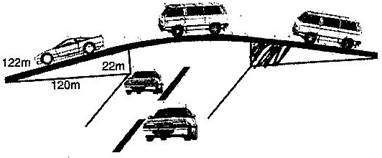Ans.
Given: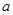= 122 m,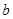= 22 m and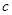= 120 m

Semi-perimeter of triangle=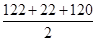=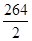= 132 m

Using Heron’s Formula,

Area of triangle =$\sqrt{132\left(132-122\right)\left(132-22\right)\left(132-120\right)}$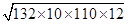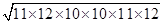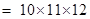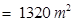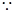Rent for advertisement on wall for 1 year = Rs. 5000 per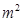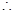Rent for advertisement on wall for 3 months for 1320 m2 =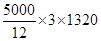= Rs. 1650000

Hence rent paid by company = Rs. 16,50,000

3. There is slide in a park. One of its side walls has been painted in some colour with a message “KEEP THE PARK GREEN AND CLEAN”, (see figure). If the sides of the wall are 15 m, 11 m and 6 m, find the area painted in colour.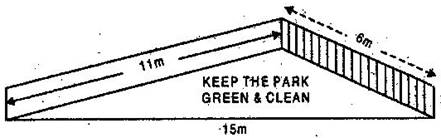Ans.
Since, sides of coloured triangular wall are 15 m, 11 m and 6 m.Semi-perimeter of coloured triangular wall =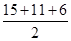=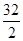= 16 m

Now, Using Heron’s formula,

Area of coloured triangular wall =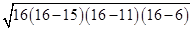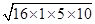=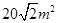Hence area painted in blue colour =4. Find the area of a triangle two sides of which are 18 cm and 10 cm and the perimeter is 42 cm.

Ans. Given:= 18 cm,= 10 cm.

Since Perimeter = 42 cm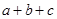=4218 + 10 += 42= 42 – 28 = 14 cmSemi-perimeter of triangle =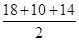= $\frac{42}{2}$= 21 cmArea of triangle =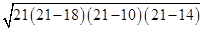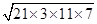=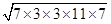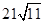= 21 x 3.3 = 69.3 cm2

5. Sides of a triangle are in the ratio of 12: 17: 25 and its perimeter is 540 cm. Find its area.

Ans. Let the sides of the triangle be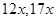and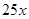.

Therefore,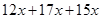= 540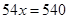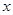= 10The sides are 120 cm, 170 cm and 250 cm.

Semi-perimeter of triangle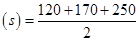= 270 cm

Now, Area of triangle =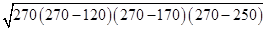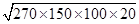=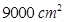6. An isosceles triangle has perimeter 30 cm and each of the equal sides is 12 cm. Find the area of the triangle.

Ans. Given:= 12 cm,= 12 cm

Since Perimeter = 30 cm=3012 + 12 += 30= 30 – 24 = 6 cmSemi-perimeter of triangle =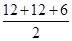= 15 cmArea of triangle =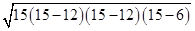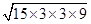=